Online Tution   »   Education News   »   CBSE Class 12 Physics Answer Key...

# CBSE Class 12 Physics Answer Key 2023, Exams Sets 1,2,3 PDF Solutions

CBSE Class 12 Physics Answer Key 2023: Students in Class 12 of the Central Board of Secondary Education (CBSE) will take the Physics exam on 6th March 2023. The CBSE Class 12 Physics exam will begin at 10:30 a.m. and end at 01:30 pm.

## Class 12 Physics Answer Key – Student’s Reaction

We talk to the examinee who appeared in the class 12 physics exam, the overall exam paper was moderate to difficult. The exam prompted a range of reactions, although most students thought it to be well-balanced. The physics exam is always difficult due to the subject’s conceptual character. Apart from a few questions that are asked every year, the questions were largely straightforward. Some numerical questions needed mathematical expertise and the application of calculus, but the others were moderate in terms of complexity.

The most scoring section of the exam was section A, which consisted of MCQs. The paper progressively grew more difficult from there. The long-answer derivations were straight from the NCERT, however, the short-answer questions had several students perplexed. Stay tuned for CBSE Class 12 Physics Answer Key 2023.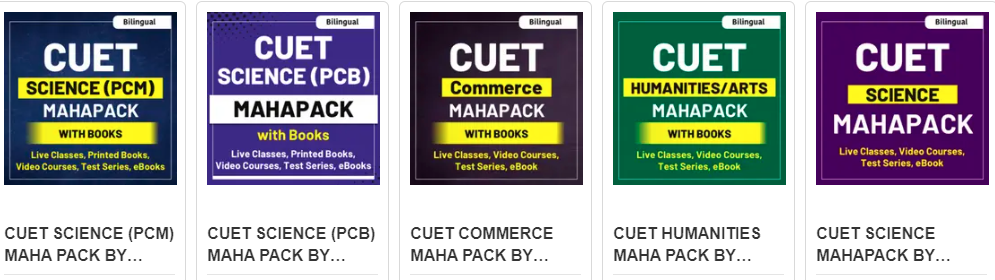## CBSE Class 12 Physics Exam Analysis 2023

The CBSE Class 12 Physics exam will be held between 10:30 and 01:30. The students can check CBSE Class 12 Physics Exam Analysis 2023 after the completion of the examination. In CBSE Class12 Physics Exam Analysis 2023, we will assess the question paper based on the errors in questions, out-of-syllabus questions, and difficulty level of the question paper.

There were no questions that were not on the syllabus, and the topic was entirely based on the NCERT textbook. The multiple-choice questions were straightforward and included a few simple numerical as well. There were roughly 18–20 numerical questions on the test, and just a few direct derivations were required. In general, more questions—including case-based questions—were based on applications.

## Class 12 Physics paper Answer Key 2023

SECTION – A

1. Two horizontal thin long parallel wires, separated by a distance r carry current I each in the opposite directions. The net magnetic field at a point midway between them will be

(A) Zero

(B) (µoI/2πr) vertically down

(C) (2µoI/r) vertically upward

(D) (µoI/πr) vertically down

2. An electric dipole of length 2 cm is placed at an angle of 30owith an electric field 2 x 105N/C. If the dipole experiences a torque of 8 x 10 -3 Nm, the magnitude of either charge of the dipole is

(A) 4 µC

(B) 7 µC

(C) 8 mC

(D) 2 mC

3. Which of the following cannot modify an external magnetic field as shown in the figure?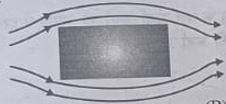(A) Nickel

(B) Silicon

(C) Sodium

(D) Copper

## Class 12 Physics Answer Key 2023 Set 1 ( 55/4/1)

Section A

Q1. Two charges q1 and q2 are placed at the centres of two spherical conducting shells of radius r1 and r2 respectively. The shells are arranged such that their centres are d [ >(r1 + r2)] distance apart. The force on q2 due to q1 is: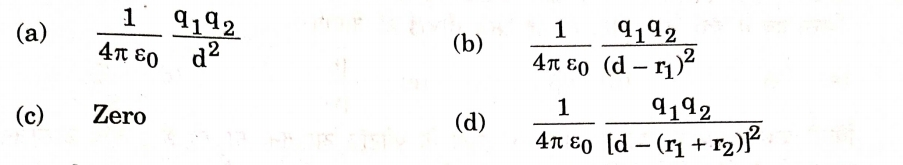Q2. An electron enters a uniform magnetic field with speed v. It describes a semicircular path and comes out of the field. The final speed of the electron is

a) zero
b) v
c) v/2
d) 2v

Q3. The magnetic field lines near a substance are as shown in the figure. The substance is: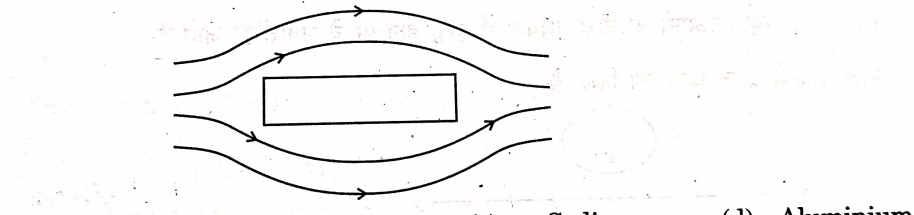a) Copper
b) Iron
c) Sodium
d) Aluminium

Q4. The figure shows the variation of current (I) with time (t) in four devices P, Q, R, and S. The device in which an alternating current flows is

a) P
b) Q
c) R
d) S

Q5. The electromagnetic waves used in radar system are-

a) Infrared waves
b) Ultraviolet rays
c) Microwaves
d) X-rays

## CBSE Class 12 Physics Exam Answer Key

 Physics Class 12 Answer Key 2023 Exam Conducting Body Central Board of Secondary Education Exam & Subject Name CBSE Class 12 Physics Category Answer Key Exam Date 6th March 2023 Unofficial Answer Key 6th March 2023 Official Answer Key To be notified Official Website https://www.cbse.nic.in/

Physics Class 12 Answer Key with Paper Solution 2023

Once the CBSE Class 12 Physics Exam 2023 will conclude, here we will provide the detailed 100% accurate CBSE Class 12 Physics Answer key 2023 with question paper analysis as soon as possible. Stay connected with us.

Class 12th Boards Ka Vardaan eBook By adda247

## Class 12 Physics Answer Key & Set 1, 2,3 Solutions

After Completion of the Exam, here we will provide the CBSE Class 12 Physics Question Papers 2023 Pdf. Candidates may download Class 12 Physics Question Papers and analyze the Class 12 Physics Answer key to calculate their expected scores.

 CBSE Class 12 Physics Question Papers 2023 Pdf Download CBSE 12 Physics Question Papers 2023- Set 1 CBSE 12 Physics Question Papers 2023- Set 2 Physics-Class-12-Question-Paper-Set-3

Expected Class 12 Physics Board Exam Paper 2023

## CBSE Class 12 Physics Question Paper Solution

Students should be searching for the right solutions to every question in the CBSE Class 12 Physics paper once the exam is done. The questions and answers are continually being drafted by Adda247 Experts. Following the conclusion of the exam, you can use the Class 12 Physics Question Paper Solution to verify all of the right answers.

Also check: CBSE Class 10 English Answer Key 2023## Class 12 Physics Answer Key 2023

Here we will give the question papers of Class 12th Physics with an answer key, match your responses with the Physics Class 12 Answer Key and sum up all the correct answers to get overall marks. As there is no negative marking so ignore the wrong answers.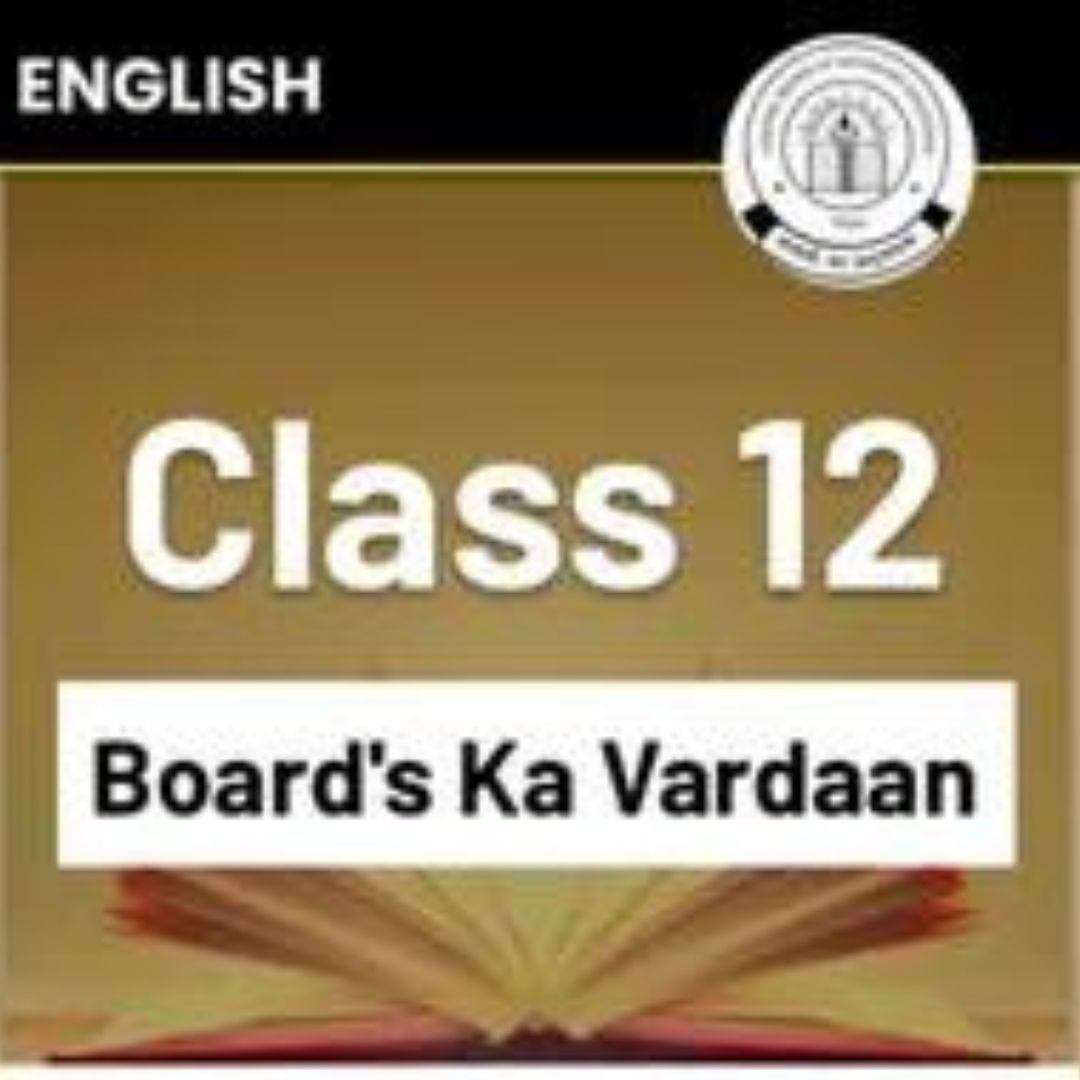## CBSE Class 12 Physics Answer key Questions and Answers- Last year

Q. A straight conducting rod of length I and mass m is suspended in a horizontal plane by a pair of flexible strings in a magnetic field of magnitude B. To remove the tension in the supporting strings, the magnitude of the current in the wire is

(a) mgB/l

(b) mgl/B

(c) mg/lB

(d) lB/mg

Q. A constant current is flowing through a solenoid. An iron rod is inserted in the solenoid along its axis. Which of the following quantities will not increase?

(a) The magnetic field at the centre

(b) The magnetic flux linked with the solenoid

(c) The rate of heating

(d) The self-inductance of the solenoidQ. A circuit is connected to an ac source of variable frequency. As the frequency of the source is increased, the current first increases and then decreases. Which of the following combinations of elements is likely to comprise the circuit ?

(a) L, C and R

(b) L and C

(c) Land R

(d) R and C

Q.  If n, e, t and m have their usual meanings, then the resistance of a wire of length l and cross-sectional area A is given by –

Q. A proton and an alpha particle move in circular orbits in a uniform magnetic field. Their speeds are in the ratio of 9:4. The ratio of radii of their circular orbits (rp/ralpha) is

(a) 3/4

(b) 4/3

(c) 8/9

(d) 9/8

Q. A coil of area 100 cm2 is kept at an angle of 30° with a magnetic field of 10-1 T. The magnetic field is reduced to zero in 10-4 s. The induced emf in the coil is –

(a) 5/3 v

(b) 5073 V

(c) 5.0 V

(d) 50.0 V

Q. A 15 2 resistor, an 80 mH inductor and a capacitor of capacitance Care connected in series with a 50 Hz ac source. If the source voltage and current in the circuit are in phase, then the value of capacitance is

(a) 100 uF

(b) 127 uF

(c) 142 uF

(d) 160 uF

Q. Four objects W, X, Y and Z, each with charge +q are held fixed at four points of a square of side d as shown in the figure. Objects X and Z are on the midpoints of the sides of the square. The electrostatic force exerted by object W on object X is F. Then the magnitude of the force exerted by object W on Z is

(a) F/7

(b) F/5

(c) F/3

(d) F/2

Q. Two sources of equal emf are connected in series. This combination is, in turn connected to an external resistance R. The internal resistance of two sources are r, and r, (r, >r,). If the potential difference across the source of internal resistance r, is zero, then R equals to

Q. Which of the following statements is correct?

(a) Magnetic field lines do not form closed loops.

(b) Magnetic field lines start from north pole and end at south pole of a magnet.

(c)The tangent at a point on a magnetic field line represents the direction of the magnetic field at that point.

(d) Two magnetic field lines may intersect each other.

Q. The equivalent resistance between A and B of the network shown in figure is

(a) 3R Ω

(b) (3/2)R Ω

(c) 2R Ω

(d) (2/3) RΩ

Q. A bar magnet has magnetic dipole moment M. Its initial position is parallel to the direction of uniform magnetic field B. In this position, the magnitudes of torque and force acting on it respectively are –

(a) 0 and MB

(b) MB and MB

(c) 0 and 0

(d) |M X B| and 0

Q. A 300 Ω resistor and a capacitor of (25/?)uF are connected in series to a 200V- 50Hz ac source. The current in the circuit is –

(a) 0.1 A

(b) 0.4 A

(c) 0.6 A

(d) 0.8 A

Q. A negatively charged object X is repelled by another charged object Y. However, an object Z is attracted to object Y. Which of the following is the most possibility for the object Z?

(a) positively charged only

(b) negatively charged only

(c) neutral or positively charged

(d) neutral or negatively charged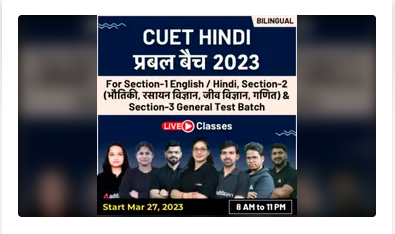Q. In an experiment, three microscopic latex spheres are sprayed into a chamber and became charged with charges +3e, +5e and -3e respectively. All the three spheres came in contact simultaneously for a moment and got separated. Which one of the following are possible values for the final charge on the spheres?

(a) +5e, -4e, +5e

(b) +6e, +6e, -7e

(c) -4e, +3.5e, +5.5e

(d) +5e, -80, +7e

Q. An object has charge of 1 C and gains 5.0 x 1018 electrons. The net charge on the object becomes –

(a) – 0.80 C

(b) + 0.80 C

(c) +1.80 C

(d) +0.20 C

Q. Kirchhoff’s first rule EI = 0 and second rule EIR = 2E (where the symbols have their usual meanings) are respectively based on –

(a) conservation of momentum and conservation of charge

(b) conservation of energy, conservation of charge

(c) conservation of charge, conservation of momentum

(d) conservation of charge, conservation of energy

Q. The electric power consumed by a 220 V – 100 W bulb when operated at 110 V is

(a) 25 W

(b) 30 W

(c) 35 W

(d) 45 W

Q. Which of the following has a negative temperature coefficient of resistivity?

(a) metal

(b) metal and semiconductor

(c) semiconductor

(d) metal and alloy

Q. Two wires carrying currents I, and I. lie. one slightly above the other, in a horizontal plane as shown in figure. The region of vertically upward strongest magnetic field is

(a) I

(b) II

(c) III

(d) IV

Q. Two parallel conductors carrying current of 4.0 A and 10.0 A are placed 2.5 cm apart in vacuum. The force per unit length between them is –

(a) 6.4 x 10-5 N/m

(b) 6.4 x 10-2 N/m

(c4.6 x 10-4 N/m

(d) 3.2 x 10-4 N/m

Q. If an ammeter is to be used in place of a voltmeter, then we must connect with the ammeter a –

(a) low resistance in parallel

(b) low resistance in series

(c) high resistance in parallel

(d) high resistance in series

Q. The magnetic field at the centre of a current-carrying circular loop of radius R, is B1. The magnetic field at a point on its axis at a distance R from the centre of the loop is B2. Then the ratio (B1/B2) is

(a) 2√2

(b) 1/√2

(c) √2

(d) 2

Q. The self-inductance of a solenoid of 600 turns is 108 mH. The self-inductance of a coil having 500 turns with the same length, the same radius and the same medium will be

(a) 95 mH

(b) 90 mH

(c) 85 mH

(d) 75 mH

Q. The rms current in a circuit connected to a 50 Hz ac source is 15 A. The value of the current in the circuit Circuit , after the instant the current is zero, is –

(a) 15/√2 A

(b) 15√2 A

(c) √215 A

(d) 8

Q. In a circuit the phase difference between the alternating current and the source voltage is Which of the following cannot be the element(s) of the circuit?

(a) only c

(b) only L

(c) L and R

(d) L and C

Q. The electric potential V at any point (x, y, z) is given by V = 3×2 where x is in metres and V in volts. The electric field at the point (1 m, 0, 2m) is –

(a) 6 V/m along -x-axis.

(b) 6 V/m along +x-axis

(c) 1.5 V/m along –x-axis

(d) 1.5 V/m along +x-axis

Q. Which of the diagrams correctly represents the electric field between two charged plates if a neutral conductor is placed in between the plates ?

Q.  A variable capacitor is connected to a 200 V battery. If its capacitance is changed from 2 uF to X uF, the decrease in energy of the capacitor is 2 x 10-2 J. The value of X is –

(a) 1 uF

(b) 2uF

(c) 3 uF

(d)) 4uF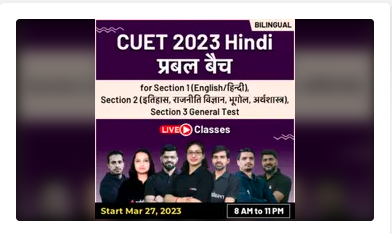Q. A potential difference of 200 V is maintained across a conductor of resistance 100 2. The number of electrons passing through it in ls is

(a) 1.25 x 1019

(b) 2.5 x 1018

(c) 1.25 x 1018

(d) 2.5 x 1016

Q. The impedance of a series LCR circuit is –

Q. When an alternating voltage E = Eo sin wt is applied to a circuit, a current I = Io sin (wt+?/2) flows through it. The average power dissipated in the circuit is

(a) Erms. Irms

(b) Eo Io

(c) Eo Io/√2

(d) Zero

Q. A current carrying wire kept in a uniform magnetic field, will experience a maximum force when it is

(a) perpendicular to the magnetic field

(b) parallel to the magnetic field

(c) at an angle of 45° to the magnetic field

(d) at an angle of 60° to the magnetic field

Q. The voltage across a resistor, an inductor, and a capacitor connected in series to an ac source are 20 V, 15 V and 30 V respectively. The resultant voltage in the circuit is

(a) 5 V

(b) 20 V

(c) 25 V

(d) 65 V

Q. In a dc circuit the direction of current inside the battery and outside the battery respectively are –

(a) positive to negative terminal and negative to positive terminal

(b) positive to negative terminal and positive to negative terminal

(c) negative to positive terminal and positive to negative terminal

(d) negative to positive terminal and negative to positive terminal

Q.  The magnitude of electric field due to a point charge 2q, at distance r is E. Then the magnitude of electric field due to a uniformly charged thin spherical shell of radius R with total charge q at a distance – (r >> R) will be

(a) E/4

(b) 0

(c) 2E

(d) 4E

Q. The horizontal component of earth’s magnetic field at a place is 0.2 G whereas it’s total magnetic field is 0.4 G. The angle of dip at the place is

(a) 30°

(b) 45°

(c) 60°

(d) 90°

Q. The current in the primary coil of a pair of coils changes from 7 A to 3 A in 0.04 s. The mutual inductance between the two coils is 0.5 H. The induced emf in the secondary coil is –

(a) 50 V

(b) 75 V

(c) 100 V

(d) 220 V

Q. A square sheet of side ‘a’ is lying parallel to XY plane at z = a. The electric field in the region is E = cz2k. The electric flux through the sheet is

(a) a4c

(b) ⅓a3c

(c) ⅓a4c

(d) 0

Q. A + 3.0 nC charge Q is initially at rest at a distance of r; = 10 cm from a + 5.0 nC charge q fixed at the origin. The charge Q is moved away from q to a new position at r, = 15 cm. In this process work done by the field is

(a) 1.29 x 10-5 J

(b) 3.6 x 105 J

(c) -4.5 x 10-7J

(d) 4.5 x 10-7 J

Q. A car battery is charged by a 12 V supply, and energy stored in it is 7.20 x 105 J. The charge passed through the battery is –

(a) 6.0 x 104 C

(b) 5.8 x 103 J

(c) 8.64 x 106 J

(d) 1.6 x 106c

Sharing is caring!

## FAQs

### When will the CBSE conduct the Physics paper for Class 12th students?

The Class 12th Physics Paper 2023 will be held on MArch 6,2023.

### How many marks does the examination in Physics in Class 12th hold?

The Physics examination for Class 12th Physics holds 70 marks.

### Does the CBSE give an official Answer Key for the Physics exams in Class 12th?

No, CBSE has not yet informed us when the official answer key for Class 12th Physics would be available. We'll keep you updated here.

### TOPICS:

•Class 12 Accounts Answer Key 2023 & ...
•IPL Schedule 2023, Start Date, Time tabl...
•Accounts Sample Paper Class 12 Accountan...
•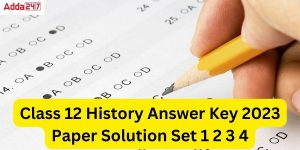Class 12 History Answer Key 2023 Paper S...
•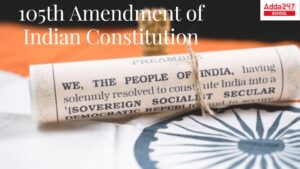105 Amendment of Indian Constitution for...
•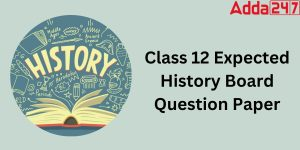Class 12 History Board Paper 2023 {Most ...

Join the Conversation

1.2.3.1.Naresh Chaurasia says:

Each question carry how many marks

2.Praphul says:

Thanks a lot for writing this, it was quite helpful and showed me a ton

3.Advika says:

Perfect blog for class 12 students. It provide ncert solution for class 12 physics subject. It is helpful for my younger brother.